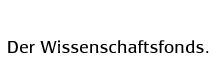P 25064Extended group analysis of differential equations> Project> Abstract> Scientists/Scholars> Project Publications> Theses> Talks> Visits> Guests> Further Activities> Cooperations> Final ReportProject publications

a) Peer-reviewed international publications

• Kurujyibwami C., Basarab-Horwath P. and Popovych R.O., Algebraic method for group classification of (1+1)-dimensional linear Schrödinger equations, Acta Appl. Math. (2018), 33 pp., arXiv:1607.04118.

• Pocheketa O.A. and Popovych R.O.,
Extended symmetry analysis of generalized Burgers equations,
J. Math. Phys. 58 (2017), 101501, 28 pp., arXiv:1603.09377.

• Opanasenko S., Bihlo A. and Popovych R.O.,
Group analysis of general Burgers�Korteweg�de Vries equations,
J. Math. Phys. 58 (2017), 081511, 37 pp., arXiv:1703.06932.

• Bihlo A. and Popovych R.O.,
Group classification of linear evolution equations,
J. Math. Anal. Appl. 448 (2017), 982�1005, arXiv:1605.09251.

• Boyko V.M., Kunzinger M. and Popovych R.O.,
Singular reduction modules of differential equations,
J. Math. Phys. 57 (2016), 101503, 34 pp., arXiv:1201.3223.

• Bihlo A., Dos Santos Cardoso-Bihlo E. and Popovych R.O.,
Invariant and conservative parameterization schemes,
Chapter 28 in Parameterization of Atmospheric Convection. Vol. 2. Current Issues and New Theories, World Scientific Publishing Co. Pte. Ltd., Hackensack, NJ, 2015, pp. 483-524.

• Bihlo A., Dos Santos Cardoso-Bihlo E.M. and
Popovych R.O.,
Algebraic method for finding equivalence groups,
J. Phys.: Conf. Ser. 621 (2015) 012001, 17 pp., arXiv:1503.06487.

• Boyko V.M., Popovych R.O. and Shapoval N.M.,
Equivalence groupoids of classes of linear ordinary differential equations and their group classification,
J. Phys.: Conf. Ser. 621 (2015) 012002, 17 pp., arXiv:1403.6062.

• Popovych D.R.,
Canonical forms for matrices of Saletan contractions,
J. Phys.: Conf. Ser. 621 (2015) 012002, 10 pp., arXiv:1507.00781.

• Vaneeva O.O., Popovych R.O. and Sophocleous C.,
Group analysis of Benjamin�Bona�Mahony equations with time dependent coefficients,
J. Phys.: Conf. Ser. 621 (2015) 012016, 13 pp. arXiv:1506.08137.

• Pocheketa O.A., Popovych R.O. and Vaneeva O.O.,
Group classification and exact solutions of variable-coefficient generalized Burgers equations with linear damping,
Appl. Math. Comput. 243 (2014) 232�244, arXiv:1308.4265.

• Vaneeva O.O., Popovych R.O. and Sophocleous C.,
Equivalence transformations in the study of integrability,
Phys. Scr. 89 (2014), 038003, 9 pp., arXiv:1308.5126.

• Bihlo A., Dos Santos Cardoso-Bihlo E. and Popovych R.O.,
Invariant parameterization and turbulence modeling on the beta-plane,
Phys. D 269 (2014), 48-62, arXiv:1112.1917.

• Dos Santos Cardoso-Bihlo E. and Popovych R.O.,
Complete point symmetry group of the barotropic vorticity equation on a rotating sphere,
J. Engrg. Math. 82 (2013), 31-38, arXiv:1206.6919.

• Pocheketa O.A. and Popovych R.O.,
Reduction operators of Burgers equation,
J. Math. Anal. Appl. 398 (2013), 270-277, arXiv:1208.0232.

• Boyko V.M., Popovych R.O. and Shapoval N.M.,
Lie symmetries of systems of second-order linear ordinary differential equations with constant coefficients,
J. Math. Anal. Appl. 397 (2013), 434-440, arXiv:1203.0387.

• Boyko V.M. and Popovych R.O.,
Reduction operators of the linear rod equation,
Proceedings of the Sixth International Workshop "Group Analysis of Differential Equations and Integrable Systems" (Protaras, Cyprus, June 17-21, 2012), University of Cyprus, Nicosia, 2013, 17-29.

• Vaneeva O.O., Popovych R.O. and Sophocleous C.,
Group classification of the Fisher equation with time-dependent coefficients,
Proceedings of the Sixth International Workshop "Group Analysis of Differential Equations and Integrable Systems" (Protaras, Cyprus, June 17-21, 2012), University of Cyprus, Nicosia, 2013, 225-237.
•

b) National publications

• Boyko V.M. and Popovych R.O.,
Conditional symmetries of the linear beam equation,
Dopov. Nats. Akad. Nauk Ukr. (2013), no. 9, 7-15. (Ukrainian)
•

c) Published theses

• Célestin Kurujyibwami,
Admissible transformations and the group classification of Schrödinger equations,
Doctoral Thesis, Linköping Studies in Science and Technology. Dissertation No. 1846, Linköping University, 2017.

• Célestin Kurujyibwami,
Group classification of linear Schrödinger equations by the algebraic method,
Licentiate Thesis, Linköping Studies in Science and Technology. Thesis No. 1743, Linköping University, 2016.

• d) Preprints

• Opanasenko S., Boyko V. and Popovych R.O.,
Enhanced group classification of nonlinear diffusion-reaction equations with gradient-dependent diffusion, 2018,
arXiv:1804.08776, 21 pp.

• Boyko V.M., Patera J. and Popovych R.O., Invariants of solvable Lie algebras with triangular nilradicals and diagonal nilindependent elements, 2018, arXiv:0706.2465v4, 21~pp. (extended and enhanced version).

• Kontogiorgis S., Popovych R.O. and Sophocleous C.,
Enhanced symmetry analysis of two-dimensional Burgers system, 2017,
arXiv:1709.02708, 32 pp.

• Opanasenko S., Bihlo A., Popovych R.O. and
Sergyeyev A.,
Extended symmetry analysis of isothermal no-slip drift flux model, 2017,
arXiv:1705.09277, 26 pp.

• Popovych R.O. and Bihlo A.,
Inverse problem on conservation laws, 2017,
arXiv:1705.03547, 26 pp.

• Dos Santos Cardoso-Bihlo E.M. and Popovych R.O.,
On the ineffectiveness of constant rotation in the primitive equations and their symmetry analysis, 2015,
arXiv:1503.04168, 15 pp.

• e) Planned publications

• Bihlo A., Dos Santos Cardoso-Bihlo E. and Popovych R.O., Invariant parameterization of geostrophic eddies in the ocean, in preparation.

• Boyko V.M., Kunzinger M. and Popovych R.O., Darboux transformation of linear evolution equations, in preparation.

• Opanasenko S. and Popovych R.O., Generalized symmetries and conservation laws of (1+1)-dimensional
Klein-Gordon equation, in preparation.

• Opanasenko S. and Popovych R.O., Admissible transformations and Lie symmetries of Kolmogorov and Fokker-Planck equations, in preparation.

• Opanasenko S., Bihlo A. and Popovych R.O., Equivalence groupoid of the class of variable-coefficient Burgers equations, in preparation.

• Kurujyibwami C., Basarab-Horwath P. and Popovych R.O., Group classification of multidimensional linear Schrödinger equations with the algebraic method, in preparation.

• Kurujyibwami C. and Popovych R.O., Group classification of multidimensional nonlinear Schrödinger equations,
in preparation.

• Kurujyibwami C. and Popovych R.O., Admissible transformations of (1+1)-dimensional Schrödinger equations with variable mass, in preparation.

• Popovych R.O. and Shevyakov A., Conservation laws of abnormal systems, in preparation.

• Vaneeva O.O., Popovych R.O. and Sophocleous C., Reduction operators and exact solutions of filtration equations, in preparation.

•With support from